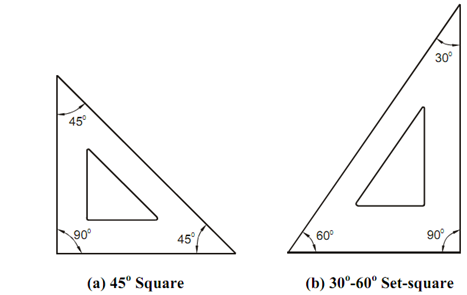## Set-square Assignment Help

Assignment Help: >> Drawing Equipment and Instruments - Set-square

Set-square:

Set-square is of triangular shape and is generally created of clear plastic. There are two kinds of set-square. They are as:

(a)  A 30o - 60o - 90o triangle

(b)  A 45o - 45o - 90o triangle

All straight lines and vertical lines in particular, except horizontal lines, can be drawn along with the assist of a set-square. During combination along with a T-square, lines inclined at 30o, 60o and 45o can be drawn. By using two set-squares along with a T-square, lines inclined at 15o, 75o and 105o can be drawn also. Parallel lines not far apart can be drawn through using of two set-squares.Figure: Set-Square